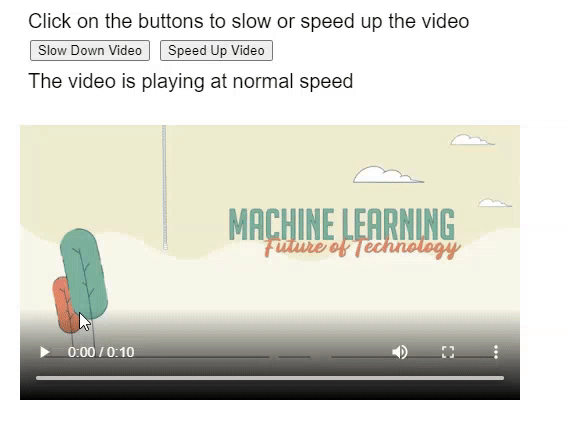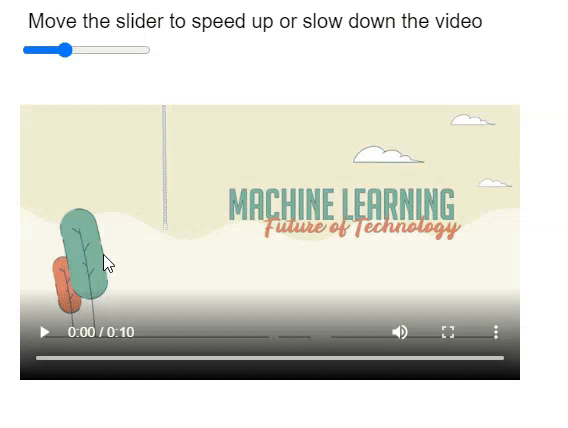# p5.MediaElement speed() Method

• Last Updated : 29 Oct, 2021

The speed() method of p5.MediaElement in p5.js is used to set or return the current playback speed of the media element. It accepts a single parameter that denotes the speed multiplier to be used for media playback. A value of 2.0 would play the media at double speed and a value of 0.5 would play it at half the speed. Negative values can also be specified to play the media in reverse, however, it may not be properly supported in all browsers.

The current playback speed of the media can be returned by not passing any parameter to the function.

Syntax:

`speed( speed )`

Parameters: This method accepts a single parameter as mentioned above and described below:

• speed: It is a number that specifies the multiplier to be used for the playback speed. It is an optional parameter.

Return Value: This method returns a Number that denotes the current playback speed of the media element.

The examples below illustrate the speed() method in p5.js:

Example 1:

## Javascript

 `function` `setup() {``    ``createCanvas(500, 400);``    ``textSize(20);` `    ``text(``"Click on the buttons to slow "` `+``         ``"or speed up the video"``, 20, 20);` `    ``example_media =``      ``createVideo(``"sample-video.mp4"``);``    ``example_media.size(500, 300);``    ``example_media.position(20, 100);` `    ``example_media.showControls();` `    ``slowBtn =``      ``createButton(``"Slow Down Video"``);``    ``slowBtn.position(30, 40);``    ``slowBtn.mousePressed(slowVideo);` `    ``fastBtn =``      ``createButton(``"Speed Up Video"``);``    ``fastBtn.position(160, 40);``    ``fastBtn.mousePressed(speedVideo);` `    ``text(``"The video is playing at normal speed"``,``         ``20, 80);``}` `function` `slowVideo() {``  ``clear();` `  ``// Slow down the video to 0.3 times``  ``// of the normal the speed``  ``example_media.speed(0.3);` `  ``text(``"The video is now slowed down"``,``       ``20, 80);` `  ``text(``"Click on the buttons to slow "` `+``       ``"or speed up the video"``, 20, 20);``}` `function` `speedVideo() {``  ``clear();` `  ``// Speed up the video to two times``  ``// the speed``  ``example_media.speed(2.0);` `  ``text(``"The video is now speed up"``,``       ``20, 80);` `  ``text(``"Click on the buttons to slow "` `+``       ``"or speed up the video"``, 20, 20);``}`

Output:Example 2:

## Javascript

 `function` `setup() {``  ``createCanvas(500, 400);``  ``textSize(20);` `  ``text(``"Move the slider to speed up "` `+``       ``"or slow down the video"``, 20, 20);` `  ``example_media =``    ``createVideo(``"sample-video.mp4"``);``  ``example_media.size(500, 300);``  ``example_media.position(20, 80);` `  ``example_media.showControls();` `  ``speedSlider =``    ``createSlider(0.1, 3.0, 1.0, 0.1);``  ``speedSlider.position(20, 40);``  ``speedSlider.input(changeSpeed);``}` `function` `changeSpeed() {``  ``clear();` `  ``let speedVal = speedSlider.value();` `  ``// Set the media speed to the slider value``  ``example_media.speed(speedVal);` `  ``// Get current playback speed``  ``let currSpeed = example_media.speed();` `  ``text(``"Move the slider to speed up or "` `+``       ``"slow down the video"``, 20, 20);``  ``text(``"Video is playing at speed: "` `+``       ``currSpeed, 20, 80);``}`

Output:Online editor: https://editor.p5js.org/
Environment Setup: https://www.geeksforgeeks.org/p5-js-soundfile-object-installation-and-methods/
Reference: https://p5js.org/reference/#/p5.MediaElement/speed

My Personal Notes arrow_drop_up